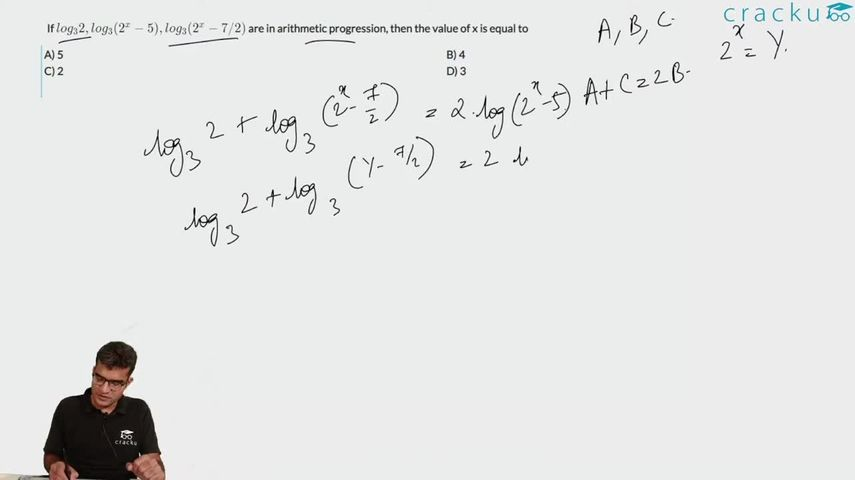Question 87

# If $$log_3 2, log_3 (2^x - 5), log_3 (2^x - 7/2)$$ are in arithmetic progression, then the value of x is equal to

Solution

$$2 log (2^x - 5) = log 2 + log (2^x - 7/2)$$
Let $$2^x = t$$
=> $$(t-5)^2 = 2(t-7/2)$$
=> $$t^2 + 25 - 10t = 2t - 7$$
=> $$t^2 - 12t + 32 = 0$$
=> t = 8, 4
Therefore, x = 2 or 3, but $$2^x$$ > 5, so x = 3

### View Video Solution